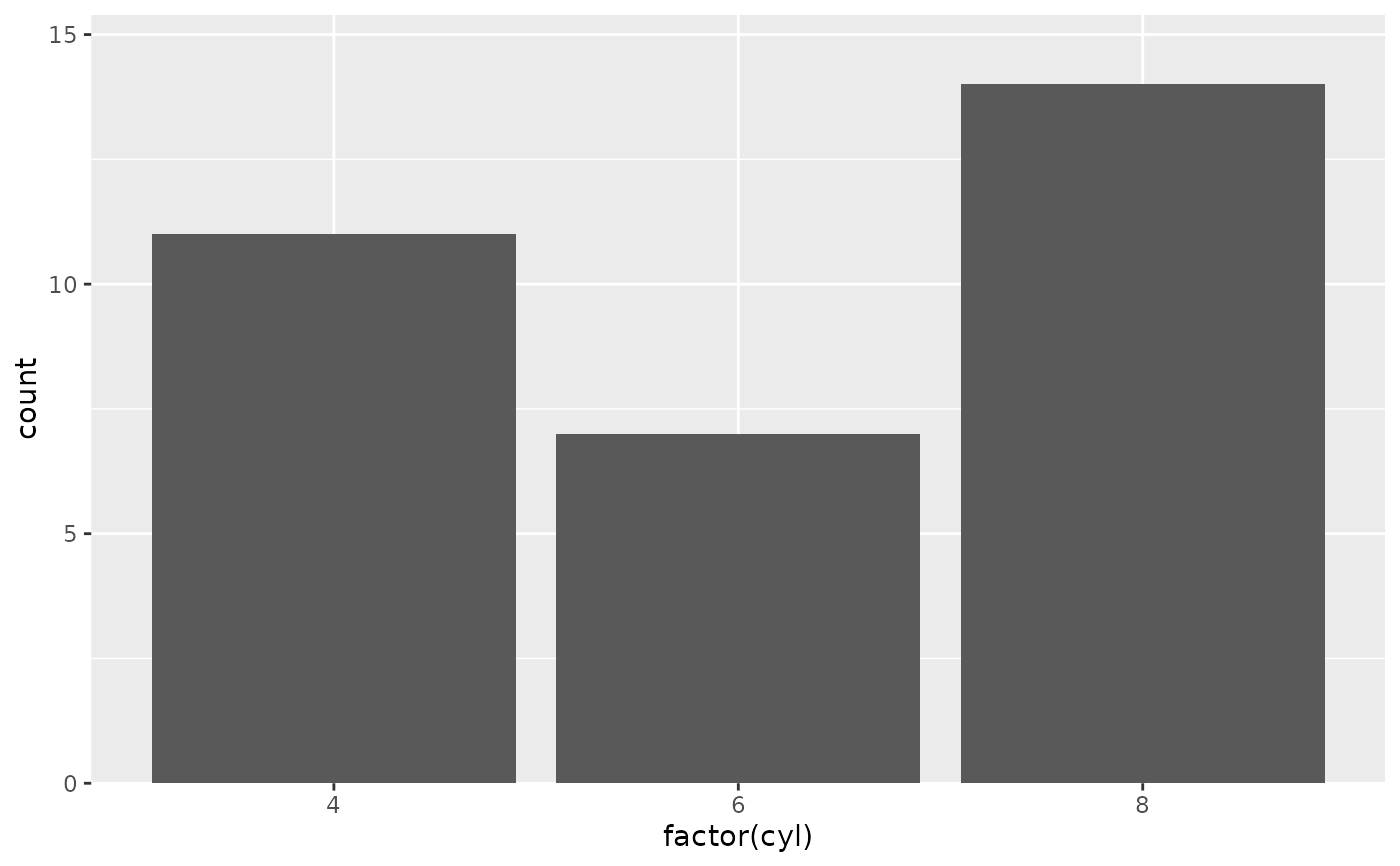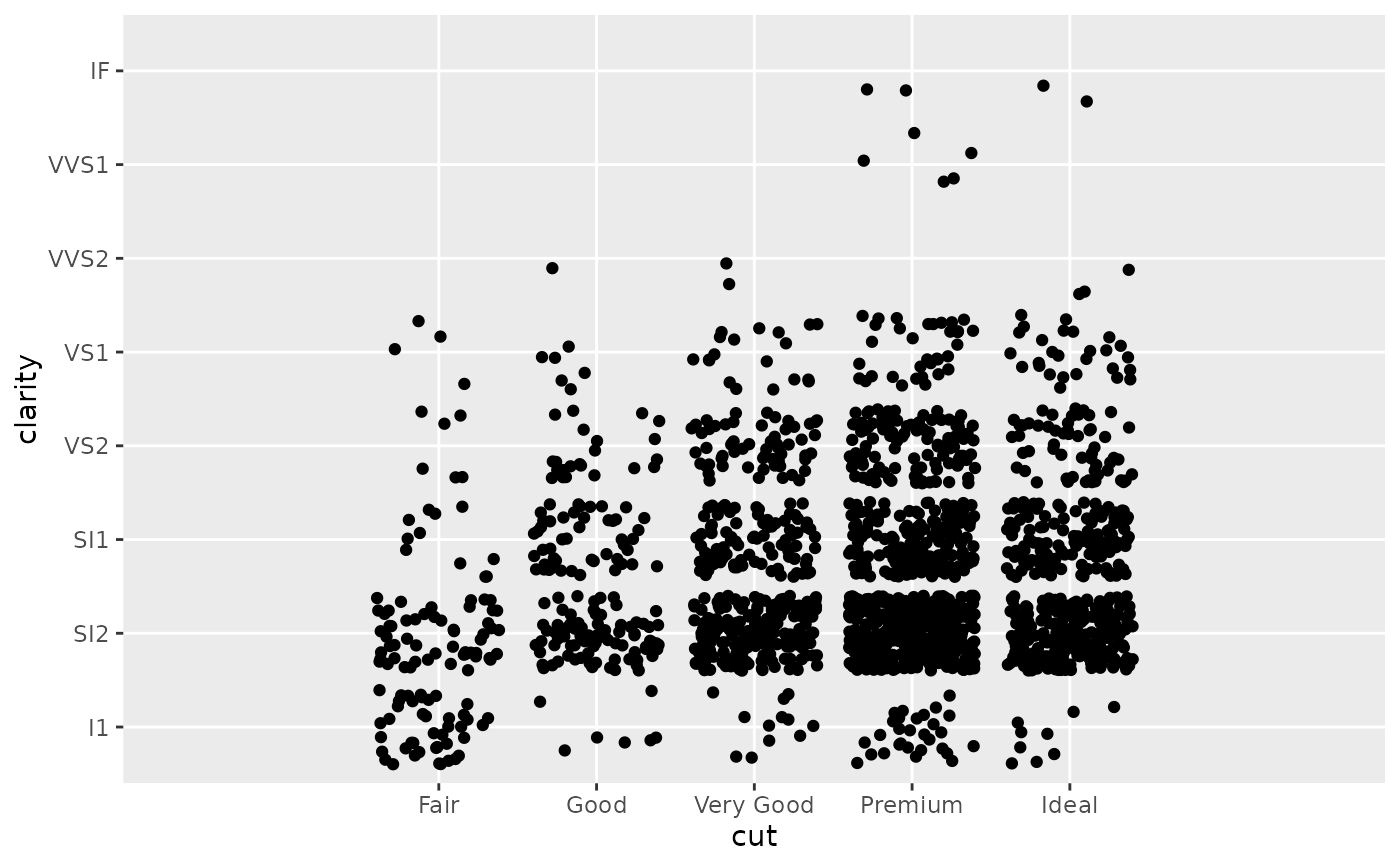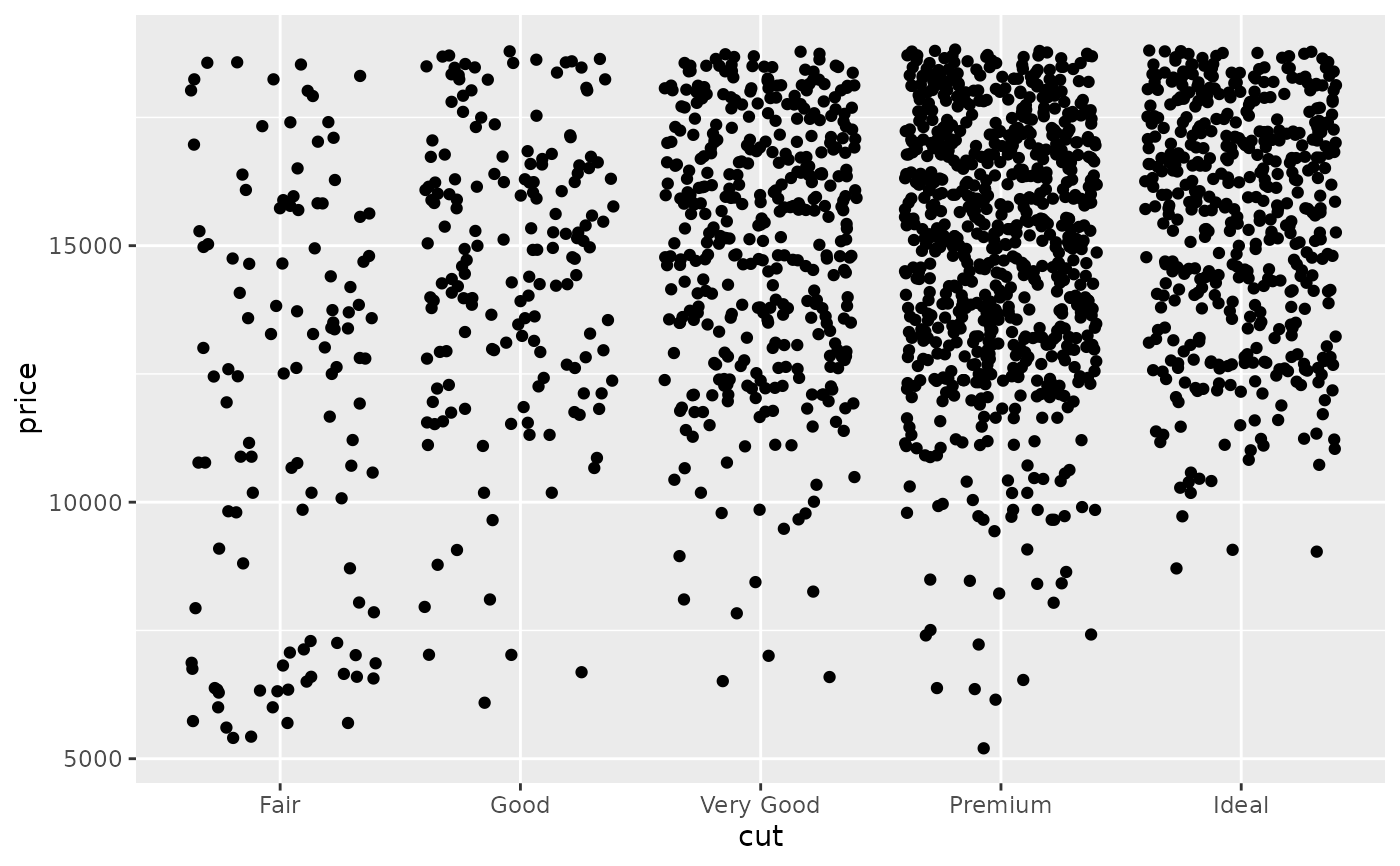This is a convenience function for generating scale expansion vectors for the expand argument of scale_(x|y)_continuous and scale_(x|y)_discrete. The expansion vectors are used to add some space between the data and the axes.

## Usage

expansion(mult = 0, add = 0)

expand_scale(mult = 0, add = 0)

## Arguments

mult

vector of multiplicative range expansion factors. If length 1, both the lower and upper limits of the scale are expanded outwards by mult. If length 2, the lower limit is expanded by mult and the upper limit by mult.

vector of additive range expansion constants. If length 1, both the lower and upper limits of the scale are expanded outwards by add units. If length 2, the lower limit is expanded by add and the upper limit by add.

## Examples

# No space below the bars but 10% above them
ggplot(mtcars) +
geom_bar(aes(x = factor(cyl))) +
scale_y_continuous(expand = expansion(mult = c(0, .1)))# Add 2 units of space on the left and right of the data
ggplot(subset(diamonds, carat > 2), aes(cut, clarity)) +
geom_jitter() +